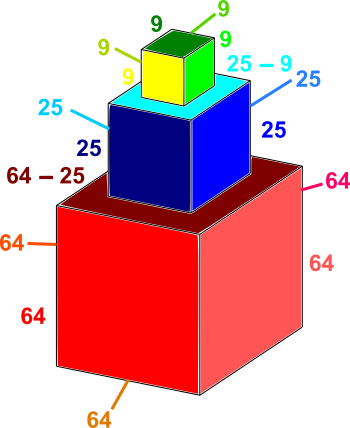You may also likeTet-trouble

Is it possible to have a tetrahedron whose six edges have lengths 10, 20, 30, 40, 50 and 60 units?Nine Colours

Can you use small coloured cubes to make a 3 by 3 by 3 cube so that each face of the bigger cube contains one of each colour?Marbles in a Box

How many winning lines can you make in a three-dimensional version of noughts and crosses?

Stacked Cubes

Age 14 to 16 ShortChallenge LevelAdding up the areas of the faces
The area of each face of each cube is found by squaring the side length.

The areas of the faces of the shape, in square centimetres, are shown on the diagram to the right.

Remember that we can't see all of the faces!

The total area is:$64 + 64+64+64+64+(64-25)+25$
$+25+25+25+(25-9)+9+9+9+9+9$
$= 64\times5 + 39 + 25\times4 + 16 + 9\times5$
$= 640\div2 + 39 + 100 + 16 + 45$
$= 320 + 139 + 61$
$= 520$

So the total surface area is $520$ cm$^2$.

Beginning with the surface area of each cube
The area of each face of each cube is found by squaring the side length.

Each cube has 6 faces, so the surface area of each cube was originally $6\times\text{side length}^2$, so the total surface area was originally $6\times8^2+6\times5^2+6\times3^2$.

However, where the largest cube joins the medium cube, the area of one face of the medium cube is lost from both cubes. Similarly, where the meduim cube joins the smallest cube, the area of one face of the smallest cube is lost from both cubes.

So the total surface area is:\begin{align}6\times8^2+6\times5^2+6\times3^2-2\times5^2-2\times3^2&=6\times8^2+4\times(3^2+5^2)\\ &=6\times64 + 4\times(9+25)\\ &=360+24 + 4\times34\\ &=360+24+120+16\\ &=480+40\\ &=520\end{align} So the total surface area is $520$ cm$^2$.
You can find more short problems, arranged by curriculum topic, in our short problems collection.